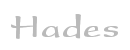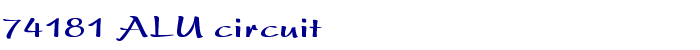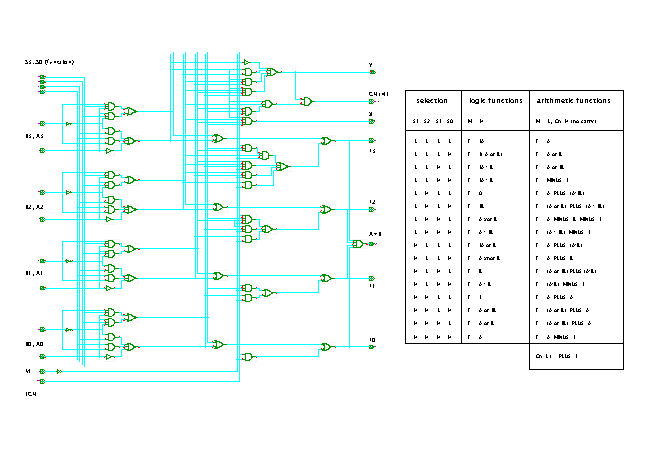TAMS / Java / Hades / applets (print version): contents | previous | next

#### TTL-series 74181 ALU circuitDescription

This circuit shows the actual implementation of the TTL-series 4-bit 74181 ALU integrated circuit. The input signals for the A and B data inputs pass through three main stages of logic.

The first stage consists of four identical blocks of logic connected to one pair of the (A,B) inputs and the four function selection inputs. Each block calculates the logical function selected by the S inputs and generates two output signals which are then passed to the next stage of logic. Note that the outputs generated by the first stage of logic are directly passed through to the F outputs (via the XOR gates) when the M input is high.

The next stage of logic realizes the carry-look-ahead calculation for the arithmetic adder functions (when M is low), while the XOR gates calculate the summation (Ai+Bi) of each pair of data inputs.

The final stage of logic consists of the output XOR gates and calculates the final sum by adding the carry bits and the (Ai+Bi) bits.

Run the applet | Run the editor (via Webstart)

Impressum | 24.11.06